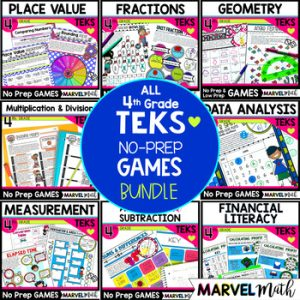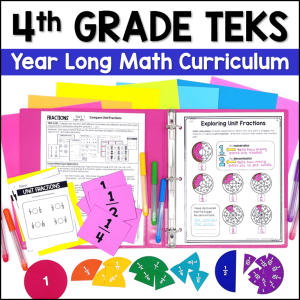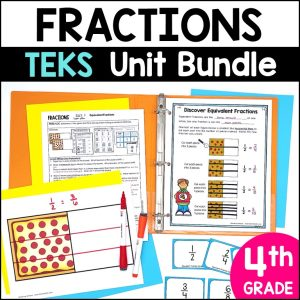Save 10% on your first order with code EXTRA10

# 4th Grade Fraction No Prep Games

\$7.00\$24.99187.25\$25.00
SKU: 3414589 Category:

## Description

This set of 11 No-Prep Fraction Games is perfect for Guided Math Stations and covers all of the 4th Grade Fraction TEKS: 4.3A, 4.3B, 4.3C, 4.3D, 4.3E, 4.3F, 4.2G, 4.3G,& 4.2H. Topics include comparing fractions, proving fractions are equivalent, fraction and decimal relationships, fractions on a number line, decomposing fractions, representing fractions as the sum of unit fractions, using benchmark fractions to estimate sums and differences, and adding and subtracting fractions.

There are 11 fun & engaging games inside and I have included both Color and Blackline versions of each.

Each game also includes a Key, so that students have a self-checking math station. Since practice makes permanent, we want to make sure they are practicing correctly!

1. Fraction & Decimal 3-in-a-row: relate decimals to fractions that name tenths and hundredths (4.2G)

2. Fraction & Decimal Bingo: decimals and fractions on number lines; relating decimals to fractions (4.2G, 4.2H, 4.3G)

3. Unit Fraction Bump: represent a fraction as the sum of unit fractions (4.3A)

4. Decompose Fractions: decompose fractions in more than one way (4.3B)

5. Equivalent Fraction Bowling: use a variety of methods to prove equivalence (4.3C)

6. Simplify Fractions to Find Equivalent Fractions (4.3C)

7. Compare Fractions: compare fractions with different numerators and denominators (4.3D)

8. Add & Subtract Fractions: word problem practice (4.3E)

9. Roll to the Top: use benchmark fractions (0, 1/4, 1/2, 3/4, 1) to estimate sums and differences (4.3F)

10. Estimate Your Treasure: use benchmark fractions to estimate in word problems (4.3E)

11. Fraction Avenue: fractions on vertical and horizontal number lines [that look like those seen in STAAR released problems] (4.3G)

This resource works seamlessly with my other 4th grade Fractions resources and is part of the comprehensive 400+page Fractions Unit & Bundle found HERE.

This is a TEKS centered resource. While others may be TEKS-aligned, they often cover Common Core standards as well. My Texas specific resources cover the TEKS and nothing but the TEKS, so that teachers dont have to spend additional time sorting through what to use and what not to use. I know how precious each and every school day is; there is no time to waste. As with all of my resources, it is designed with STAAR in mind.